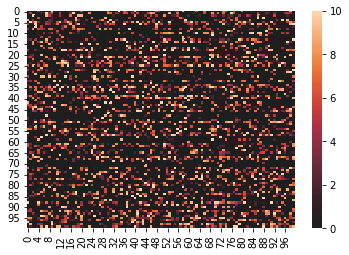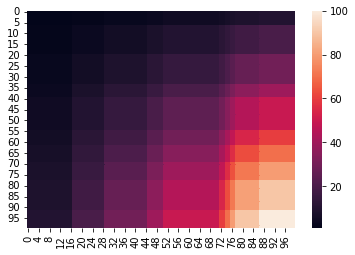My roles in data analytics/science so far have always been focused on online marketing applications, such as analyzing the impact of ad campaigns, the engagement of users with a website, or the performance of blog posts. Machine Learning algorithms and Python libraries like scikit-learn can help marketers derive insights from user data and establish connections between their features or actions that otherwise would go unnoticed.

In my search for resources that bridge data science and marketing, I found the chapter Clustering and Unsupervised Models for Marketing$$^1$$, which explains how to use spectral biclustering for making product recommendations. In this post, I share with you my learnings about this algorithm and its implementation in Python, as taken from the book.

## Biclustering methods

Biclustering is a clustering method that concomitently operates on two levels that are correlated by the presence of a medium (e.g., customers and products by rating). Biclustering aims to find the regions where the medium is cohesive (e.g., the rating is high or low) by rearranging the structure of both levels.

Biclustering operates on a matrice $$A ∈ R^{n \times m}$$. In a marketing example, the rows and columns can represent customers and products. Each element $$a_{ij} ∈ A$$ indicates a rating (or zero) for the product $$p_j$$ given by the customer $$c_i$$. $$A$$ has an underlying checkerboard structure, where the compact regions (biclusters) represent sub-matrices.

## Theory: Spectral Biclustering algorithm

Spectral Biclustering is an algorithm developed by Kluger et al. (2003) for classifying genes and conditions. Spectral Biclustering relies on Singular Value Decomposition (SVD) and was initially applied to bioinformatics tasks, but has found applications in other fields as well.

The algorithm consists of five steps:

1. Bistochaization, i.e. a preprocessing iterative phase where the values of $$a_{ij}$$ are adjusted so that all row and column sums add up to a constant common value (usually 1).
2. Decomposition of the bistochastic matrix $$A_b$$ using SVD. $$A_b$$ is decomposed into left and right singular vectors ($$A_bA_b^T$$ and $$A_b^TA_b$$). The singular values are sorted in descending order and the singular vectors are rearranged accordingly.
3. Ranking of the singular vectors by analyzing their similarity with $$\bar{v}_p$$, the checkerboard structure to be highlighted by biclustering.
4. Projection of the data set onto the sub-space spanned by the columns of $$P_k$$.
5. Application of K-Means to find the labelling for the $$k$$ clusters. This operation yields two label vectors, $$\bar{r}$$ and $$\bar{c}$$.

## Practice: Spectral Biclustering for marketing

To implement spectral biclustering, we first need a data set. For practical reasons, we can generate an artificial data set that consists of:

• 100 users
• 100 transactions (purchases)
• 100 products
• ratings in the range 1-10 (10 different possible biclusters)
import numpy as np

nb_users = 100
nb_products = 100

items = [i for i in range(nb_products)]
transactions = []
ratings = np.zeros(shape=(nb_users, nb_products), dtype=np.int)

for i in range(nb_users):
n_items = np.random.randint(2, 60)
transaction = tuple(np.random.choice(items, replace=False, size=n_items))
transactions.append(list(map(lambda x: "P{}".format(x+1), transaction)))

for t in transaction:
rating = np.random.randint(1, 11)
ratings[i, t] = rating


We can visualize the generated ratings on a heatmap:

import seaborn as sns
sns.heatmap(ratings, center=0)Now we can implement the spectral biclustering algorithm with scikit-learn:

from sklearn.cluster.bicluster import SpectralBiclustering

sbc = SpectralBiclustering(n_clusters = 10,
n_best = 5, #project the dataset on the top 5 singular vectors
svd_method = "arpack", #good for small/medium matrices
n_jobs = -1,
random_state = 1000)

sbc.fit(ratings)


Next, we need to compute the final matrix using the outer product of the sorted row and column indices:

rc = np.outer(np.sort(sbc.row_labels_) + 1,
np.sort(sbc.column_labels_) + 1)


We can visualize the rearranged matrix on a heatmap as well:

sns.heatmap(rc)Finally, we can use the matrix for a practical marketing use case: determining the group of users that rated a group of five products in order to send those users a newsletter with product recommendations. To do this, we need to select all the rows and columns associatd with the biclusters with an index of 5 (because 0 means no rating).

print("Users: {}".format(np.where(sbc.rows_[8, :] == True)))
print("Products: {}".format(np.where(sbc.columns_[8, :] == True)))


The code above outputs the following result:

Users: (array([ 7, 26, 30, 44, 54, 66, 95]),)
Products: (array([61, 64]),


This means that we need to check the products in the Products array, select other products that are similar to those, and send them to the users in the Users array.

There you have it–Spectral Biclustering implemented in Python with scikit-learn for marketing recommendations.

1. Bonaccorso, G. (2020). Clustering and Unsupervised Models for Marketing. In: Mastering Machine Learning Algorithms, 233–246. UK: Packt Publishing.
2. Kluger, Y. et al. (2003). Spectral biclustering of microarray data: coclustering genes and conditions. Genome research, 13(4), 703–716.